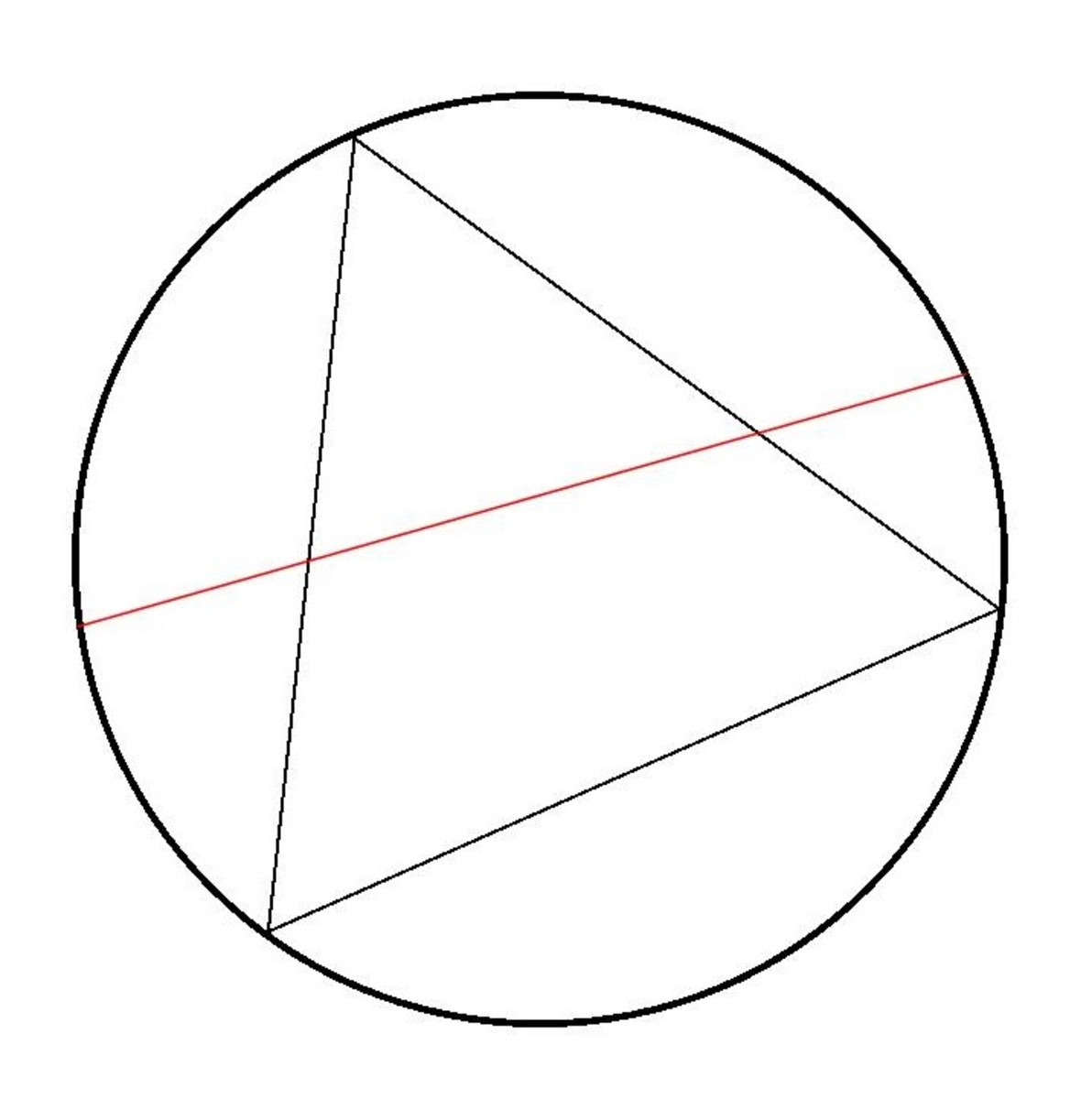# Bertrand's Paradox— A Problem in Probability Theory

• Author:
• Updated date:

I am a former maths teacher and owner of DoingMaths. I love writing about maths, its applications, and fun mathematical facts.

## What Is Bertrand's Paradox?

Bertrand's Paradox is a problem within probability theory first suggested by the French Mathematician Joseph Bertrand (1822–1900) in his 1889 work 'Calcul des Probabilites'. It sets a physical problem that seems to be very simple, but that leads to differing probabilities unless its procedure is more clearly defined.A circle with an inscribed equilateral triangle and a chord

Look at the circle in the picture above containing an inscribed equilateral triangle (i.e. each corner of the triangle lies on the circumference of the circle).

Suppose a chord (a straight line from circumference to circumference) is drawn randomly on the circle, such as the red chord in the diagram.

What is the probability that this chord is longer than a side of the triangle?

This seems like a reasonably simple question that should have an equally simple answer; however, there are actually three different answers depending upon how you 'randomly choose' the chord. We will look at each of these answers here.

### Three Ways to Randomly Draw a Chord on a Circle

1. Random Endpoints
3. Random Midpoint

## Solution 1: Random Endpoints

In solution 1, we define the chord by randomly choosing two endpoints on the circumference and joining them together to create a chord. Imagine the triangle is now rotated to match up one corner with one end of the chord as in the diagram. You can see from the diagram that the other endpoint of the chord decides whether this chord is longer than the triangle edge or not.

Chord 1 has its other endpoint touching the circumference of the arc between the two far corners of the triangle and is longer than the triangle sides. Chords 2 and 3, however, have their endpoints on the circumference between the start point and the far corners, and it can be seen that these are shorter than the triangle sides.

It can be seen quite easily that the only way that our chord can be longer than a triangle side is if its far endpoint lies on the arc between the far corners of the triangle. As the triangle's corners split the circle's circumference into exact thirds, there is a 1/3 chance that the far endpoint sits on this arc; hence, we have a probability of 1/3 that the chord is longer than the triangle's sides.

Scroll to Continue

## Solution 2: Random Radius

In solution 2, rather than define our chord by its endpoints, we instead define it by drawing a radius on the circle and constructing a perpendicular chord through this radius. Now imagine rotating the triangle so that one side is parallel to our chord (hence also perpendicular to the radius).

We can see from the diagram that if the chord crosses the radius at a point closer to the circle's centre than the side of the triangle (like chord 1), then it is longer than the triangle's sides, whereas if it crosses the radius closer to the circle's edge (like chord 2), then it is shorter. By basic geometry, the triangle's side bisects the radius (cuts it in half), so there is a 1/2 chance that the chord sits nearer to the centre, hence a probability of 1/2 that the chord is longer than the triangle's sides.

## Solution 3: Random Midpoint

For the third solution, imagine that the chord is defined by where its midpoint sits within the circle. In the diagram, there is a smaller circle inscribed within the triangle. It can be seen in the diagram that if the midpoint of the chord falls within this smaller circle, like Chord 1's does, then the chord is longer than the triangle's sides.

Conversely, if the chord's centre lies outside of the smaller circle, then it is smaller than the triangle's sides. As the smaller circle has a radius 1/2 the size of the larger circle, it follows that it has 1/4 of the area. Therefore there is a probability of 1/4 that a random point lies within the smaller circle, hence a probability of 1/4 that the chord is longer than a triangle side.

## But Which Answer Is Correct?

So there we have it. Depending upon how the chord is defined, we have three completely different probabilities of it being longer than the triangle's edges; 1/4, 1/3 or 1/2. This is the paradox that Bertrand wrote about. But how is this possible?

The problem comes down to how the question is stated. As the three solutions given refer to three different ways of randomly selecting a chord, they are all equally viable solutions, hence the problem, as originally stated, does not have a unique answer.

These differing probabilities can be seen physically by setting up the problem in different ways.

Suppose you defined your random chord by randomly selecting two numbers between 0 and 360, placing points this number of degrees around the circle and then joining them up to create a chord. This method would lead to a probability of 1/3 that the chord is longer than the triangle's edges as you are defining the chord by its endpoints as in solution 1.

If, instead, you defined your random chord by standing at the side of the circle and throwing a rod across the circle perpendicular to a set radius, then this is modelled by solution 2, and you will have a probability of 1/2 that the chord created will be longer than the triangle's sides.

To set up solution 3, imagine something was thrown in a completely random fashion into the circle. Where it lands marks the midpoint of a chord, and this chord is then drawn accordingly. You would now have a probability of 1/4 that this chord will be longer than the triangle's sides.